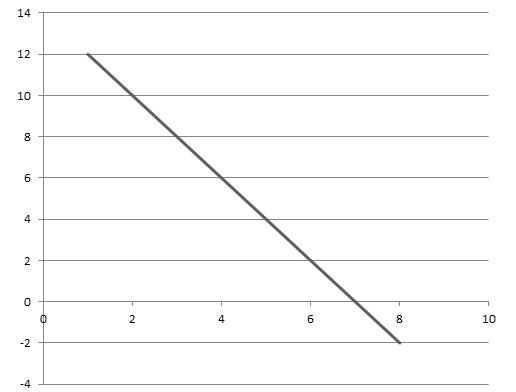# The Law of diminishing marginal utility

This law was first developed by H.H Gossen in 1854 AD, which is also the first law of Gassen. This law is based on universal human experience. It explains that for more units of commodity; its M.U derived from each additional unit diminishes in comparison to the previous unit. Hence, the law of diminishing marginal utility implies that consumption of each successive units of a particular commodity gives less and lesser satisfaction to the consumer if a consumer consumes it in a certain time period.

This law is based on the following assumptions:

1. The consumption is rational
2. Price of the commodity remains constant
3. Income of the consumers remains constant
4. There is no any time gap between the consumption of different units
5. Size of commodity is suitable.

We can explain this law by the help of given table and figure:

 Units of commodity M.U 1 12 2 10 3 8 4 6 5 4 6 2 7 0 8 -2

On the above table, when the consumer consumes one unit of commodity then marginal utility is 12. When consumption of commodities gradually increases from 1 to 8, the marginal utility decreases from 12 to -2. When consumer consumes 7 units then at that time marginal utility is 0. We can explain the same table by the help of following figure:On the above graph x and y-axes measures units of commodity and M.U respectively. Marginal utility curve is derived on the basis of above table which is negatively slopped and tends to negative. It indicates when consumer consumes more and more commodity M.U starts to decline and it tends to the negative.